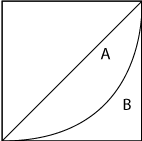Gini coefficient is an indicator which measures the level of income equality from the Lorenz curve. As illustrated in the below diagram, assuming A represents the area between the curve of the actual income distribution and the line of complete income equality, and B is the area beneath the curve of the actual income distribution, the quotient of A/(A+B) indicates the extent of inequality, which is known as the Gini coefficient or the Lorenz coefficient.

The value of Gini coefficient varies between 0, which reflects complete income equality, and 1, which signifies complete inequality of income distribution. When income distribution moves towards equality, the arc of the Lorenz curve tends to flatten and value of the Gini coefficient is closer to 0. On the contrary, when income distribution moves towards inequality, the arc of the Lorenz curve tends to bow further and value of the Gini coefficient is closer to 1.